• 代码 #include #include int main() { int m = 0,n =0,start = 0,end = 0,flag =0; float temp =0.0; scanf("%d",&m); scanf("%d",&n); temp = (float)m/n-(float)(n-1)/2;... if(temp==(in
代码

#include <stdio.h>
#include <math.h>
int main()
{
int m = 0,n =0,start = 0,end = 0,flag =0;
float temp =0.0;
scanf("%d",&m);
scanf("%d",&n);
temp = (float)m/n-(float)(n-1)/2;
if(temp==(int)temp)
{
for(flag =1,start =(int)temp,end = start+n;start<end;start++)
printf("%d",start);
printf("\n");
}
if(flag==0)
printf("没有符合条件的数\n");
return 0;
}

展开全文• 为了计算一个正整数中二进制表示1的个数。 我们可以采用查表的办法。 下面四位四位的计算： 0000对应0个1 0001对应1 个1 0010 对应1个1 .................... 1110 对应3个1 1111对应4个1 所以我们建立如下size为16...
为了计算一个正整数中二进制表示1的个数。 我们可以采用查表的办法。
下面四位四位的计算：
0000对应0个1
0001对应1 个1
0010 对应1个1
....................
1110 对应3个1
1111对应4个1
所以我们建立如下size为16的数组用于映射0， 1， 2， 3， ....., 15这16个数字对应的整数1的个数。
#include <iostream>

using namespace std;
static int numBits[] = {0, 1, 1, 2,
1, 2, 2, 3,
1, 2, 2, 3,
2, 3, 3, 4};

int bitsCount(unsigned int n) {
int bitNumber = 0;
/*
// 方法二:
for(; n!= 0; n >>= 4) {
bitNumber += numBits[n & 0x0f];
}
*/
do {
bitNumber += numBits[n & 0x0f];

} while(n>>=4); // 注意必须是n>>=4， 不要忘了等号
return bitNumber;
}

int main() {
cout << bitsCount(15) << endl;
return 0;
}



运行结果如下：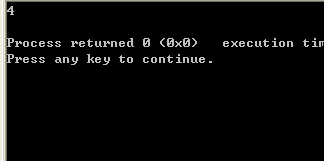方法二： 又被称为Brian Kernighan's algorithm
也就是我们从给定的测试的数减去1， 将会toggles all the bits(from right to left) till the rightest set bit(including the rightestmost set bit)。 so if we subtract a number by a, and do bitwise & with itself(n &(n - 1))， we unset the rightmost set bit. if we do n&(n-1) in a loop
and count the number of times loop executes we get the set bit count. 伪代码如下：
1  Initialize count: = 0
2  If integer n is not zero
(a) Do bitwise & with (n-1) and assign the value back to n
n: = n&(n-1)
(b) Increment count by 1
(c) go to step 2
3  Else return count
程序如下：
#include <cstdio>
int countSetBits(int n) {
unsigned int c = 0;
while(n) {
n &= (n-1) ;
c++;
}
return c;
}

/* Program to test function countSetBits */
int main() {
int i = 9;
printf("%d", countSetBits(i));
return 0;
}

运行如下：


展开全文• 已知正整数n的立方可以表示为n个连续奇数的和，例如： 3的3次方 = 7 + 9 + 11 （不会打那个次方数） 6的3次方 = 31 + 33 + 35 + 37 + 39 + 41 对于任意给定的正整数m，如何得到这连续m个奇数呢？企业应用
• 如何判断并求出一个整数x是否可以表示成n（n>=2）个连续正整数的和，可以借鉴
理论分析：因为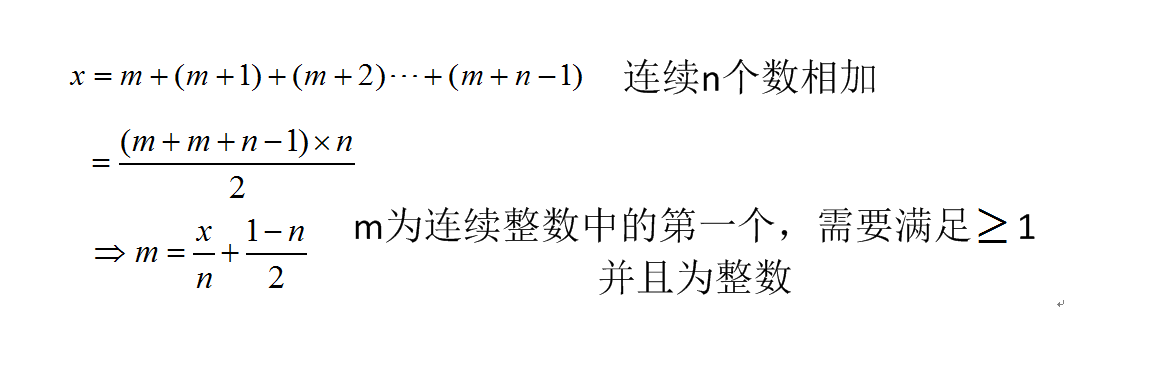#include <iostream>
#include <vector>
using namespace std;
int  main()
{int m;while(cin>>m){vector<vector<vector<int>>> alll;for (int i=2;i<m;i++){//int i=m;vector<vector<int>> all;for (int j=2;j<i/2;j++){ vector<int> al;float temp=float(i*1.0/j)+float((1-j)*1.0/2);if (temp>=1.0){int temp_int=int (temp);if (temp-temp_int<0.00001){al.push_back(i);for (int k=temp_int;k<j+temp_int;k++){al.push_back(k);}}}if (!al.empty())all.push_back(al);}if (!all.empty())alll.push_back(all);}for (int kk=0;kk<alll.size();kk++){cout<<alll[kk]<<" :";for (int jj=0;jj<alll[kk].size();jj++){cout<<"\t";for (int ii=1;ii<alll[kk][jj].size();ii++)cout<<alll[kk][jj][ii]<<" ";cout<<endl;}cout<<"--------------------------------------------"<<endl;}}return 0;
}

展开全文算法 CC++
• 今天给大家讲一下有关数制、码制、基本逻辑运算等数字电路基本知识。这些知识贯穿在所有的数字电子技术中，包括工业自动化...数制的知识要求掌握2、10、16进制数的表示和它们相互间的转换。码制的知识要求掌握各种...
今天给大家讲一下有关数制、码制、基本逻辑运算等数字电路基本知识。这些知识贯穿在所有的数字电子技术中，包括工业自动化控制技术，例如；PLC、单片机、单板工控机、变频器、伺服、步进等控制技术中，我们要求大家一定要学好这些基本知识及其应用。学习这些知识不需要高深的数理知识，初中以上水平就可以理解掌握，只要努力学习就行。数制的知识要求掌握2、10、16进制数的表示和它们相互间的转换。码制的知识要求掌握各种码制的特点及其应用。基本逻辑运算知识要求掌握基本逻辑运算的关系，表示及与电路间的联系。一、数制数制，就是数的计数方法，也就是数的进位法。在数字电子技术中，数制是必须掌握的基础知识。二、数制三要素数制是指计算数的方法。其基本内容有二个，一个是如何表示一个数，一个是如何表示数的进位。公元400年，印度数学家最早提出了十进制计数系统，当然，这种计数系统与人的手指有关。这也是很自然的事，这种计数系统(就是数制)的特点是逢十进一，有10个不同的数码表示数(也就是0～9个阿拉伯数字)，我们把这个计数系统叫做十进制。十进制计数内容已经包含了数制的三要素：基数、位权、复位和进位。下面我们就以十进制为例来讲解数制的三要素。下面是一个十进制表示的数：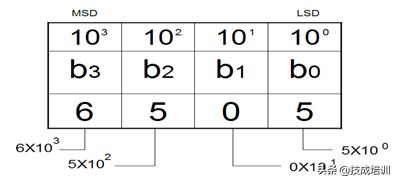这是一个十进制的数：6505，是一个四位数。其中，6、5、0是它的数码，也叫数符。我们知道：十进制数有10个数码。0～9。我们把这10个数码叫做10进制数的基数。基数即表示了数制所包含数码的个数，同时也包含了数制的进位，即逢十进一。N进制必须有n个数码。我们把这四位数的位分别以b0位，b1位，b2位，b3位表示数码所在的位。(也即我们日常所说的个位，十位，百位，千位)。注意：我们规定最右位(个位)为b0位，然后依次往左为b1，b2。。。。。。位。我们会发现b2位的5和b0位的5虽然都是数码5，但他们表示的数值是不一样的。b2位的5表示500，b0位的5只表示5，为什么呢？这是因为不同的位的位值是不一样的，位值又叫位权。位权是数制的三要素之一，它表示数码所在位的值。位权一般是基数的正整数幂，从0开始，按位递增。b0位位权为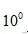，b1位位权为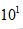。。。以次类推。N进制的位权为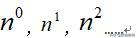当数中某一位(例如b0位)到达最大数码值后，必须产生复位和进位的运转。当b0数到9(最大数码)后则b0位会变为0.并向b1位进1。复位和进位是数制必须的运算处理。我们把基数，位权，进位和复位称之为数制三要素。一般地说，数制的数值由各位数码乘以位权然后相加得到。即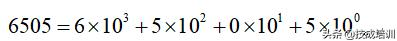我们把数制中数的位权最大的有效值(最左边的位)叫做最高有效位MSD(Most Siginfical Digit)。而把最右边的有效位叫做最低有效位LSD(Least Siginfical Digit)。在二进制中，常常把LSD位叫做低位。而把MSD位叫高位。上面虽然是以十进制来介绍数制的知识的，但是数制的三要素对所有的进制都是适用的。一个N进制的n位数，则：基数为N，有n个不同的数码，逢N进一，其位权由LSD位到MSD位分别为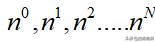当某位计数到最大数码时，该位复位为最小数码，并向上一位进1，而其数值为：数值=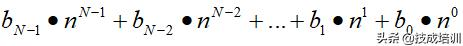往期优秀文章回顾：一个电气新手，我想问PLC电气编程用什么电脑最好？
展开全文• 给定一个正整数如何判断它是否是一段连续正整数之和？ 比如，给定正整数 15，它可以表示成 15 = 1 + 2 + 3 + 4 + 5，也可以表示成 15 = 7 + 8。 给定正整数 6，它可以表示成 6 = 1 + 2 + 3。 那么这样的正整数有...
• Problem Description The game of gizilch has very simple rules. First 100 grapes are labeled, in nontoxic ink, with the numbers 1 to 100. Then, with a cry of GIZILCH!'', the referee fires the grapes...Golang erlang r语言
• 题目：如何判断一个整数 x 是否可以表示成 n（n >= 2）个连续正整数的和。 思路分析： （1）假设 x 可以表示成 n（n >= 2）个连续正整数的和，那么数学表达式如下：x = m + (m + 1) + (m + 2) + … + (m + n - 1)...整数分解
• 如判断一个正整数是否为素数，则要用到带else字句的循环结构。 代码如下 n = int(input(“输入一个正整数n（n>=2）：”)) for i in range(2,n): if n%i==0: print(n,“不是素数”) break else: print(n,“是素数...python
• 展开全部 import java.io.BufferedReader;... } } 运行后的内容： 请输入要输入的正整数个数： 6 请输入正整数，每个正整数之间用","分割： 876,234,123,2,32,12 冒泡排序后的数组内容： 2,12,32,123,234,876,
• 实现一个方法，判断一个正整数是否是2的乘方（比如16是2的4次方，返回True；否则返回False） 方法一：从1开始循环乘以2，直到结果第一次大于或者等于目标值，如果相等，则放回True，如果大于，则返回False 方法二：...
• var reg = new RegExp("^[0-9]*[1-9][0-9]*$"); var flag=reg.test(num); flag 为 true，表示输入为正整数正则 js • 下面以“取给定正整数的指定bit位开始的指定长度的数据”为例说明如何将整数拆分成bit位表示的形式。 取给定正整数的指定bit位开始的指定长度的数据。bit位从右向左从0开始计算。 函数原型： unsigned int ...c语言 • 题目：输入一个正整数，若该数能用几个连续正整数之和表示，则输出所有可能的正整数序列。一个正整数有可能可以被表示为n(n>=2)个连续正整数之和，如：15=1+2+3+4+515=4+5+615=7+8有些数可以写成连续N(>1)个... • 问题描述官网题目地址：力扣​leetcode-cn.com问题...首先最容易想到的应该就是 hash，因为把所有正整数往里面一丢，再一个一个查就可以了。但是题目要求不能有额外的空间开销。如果使用hash map的，空间开销为 O(... • 正整数的十进制转换二进制将一个十进制数除以二，得到的商再除以二，依此类推直到商等于一或零时为止，倒取除得的余数，即换算为二进制数的结果。只需记住要点：除二取余，倒序排列。由于计算机内部表示数的字节单位... • 问题:如何快速把$cos^4xsin^3x$表示成正弦，余弦的线性组合？ 分析:利用牛顿二项式展开以下表达式: 再利用欧拉公式$e^{i\theta}=cos\theta+isin\theta\$ 比如： 解答: 评:这样的变换,表示成线性组合在求...
• 题目：输入一个正整数，若该数能用几个连续正整数之和表示，则输出所有可能的正整数序列。 一个正整数有可能可以被表示为n(n>=2)个连续正整数之和，如： 15=1+2+3+4+5 15=4+5+6 15=7+8 有些数可以写成连续N...
• x = 2^k 当且仅当 (x&(x-1) == 0) ...把x表示为2进制形式，首先因为x不为0，所以1的位数至少为1位；又因为x ！= 2^k，所以1的位数至少为2位；则最高位的1在x和x-1中都必然存在，故x&(x-1) ！= 0。
• 题目：输入一个正整数，若该数能用几个连续正整数之和表示，则输出所有可能的正整数序列。 一个正整数有可能可以被表示为n(n>=2)个连续正整数之和，如： 15=1+2+3+4+5 15=4+5+6 15=7+8 有些数可以写成连续N（>1）个...
• Zeus 和 Prometheus 做了一个游戏，Prometheus 给 Zeus 一个集合，集合中包含了N个正整数，随后 Prometheus 将向 Zeus 发起M次询问，每次询问中包含一个正整数 S ，之后 Zeus 需要在集合当中找出一个正整数 K ，使得...Golang erlang r语言
• 题目：输入一个正整数，若该数能用几个连续正整数之和表示，则输出所有可能的正整数序列。一个正整数有可能可以被表示为n(n>=2)个连续正整数之和，如：15=1+2+3+4+515=4+5+615=7+8有些数可以写成连续N(>1)个...分解连续自然数的和...